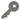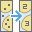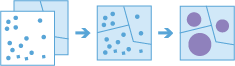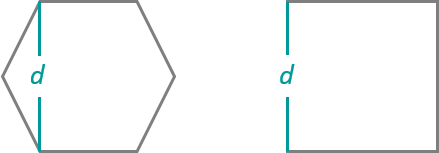# Aggregate PointsAvailable in big data analytics.

The Aggregate Points toolcan collect and summarize point features within area features. The boundaries from the area features are used to collect the points within each area and they are then used to calculate statistics. The resulting layer contains the count of points within each area as well as summary calculations.

## Workflow diagram## Example

Tornadoes are a violent type of storm that occurs in the United States. You want to know the effect of tornadoes, including loss of life, injuries, property damage, and financial loss, in each state and county. You have access to tornado locations, but you need a better way to visualize the data within certain areas. You can aggregate your tornado data into state and county boundaries and normalize your data by population to find the areas most affected by tornadoes.

## Usage notes

• The output layer is always a polygon layer. Only the polygon bins or features where points occur are returned.
• You can provide the area layer to use for the analysis, or bins can be generated of a specified size and shape (hexagon or square), into which point features are aggregated. The bin size specifies how large the bins are. If you are aggregating into hexagons, the size is the height of each hexagon and the width of the resulting hexagon will be 2 times the height divided by the square root of 3. If you are aggregating into squares, the bin size is the height of the square, which is equal to the width.• If time is enabled on your input layer, you may apply time stepping to your analysis. For details, see How time stepping works.
• The most basic aggregations will calculate a count of the number of points in each area. Statistics (count, sum, minimum, maximum, range, mean, standard deviation, and variance) can also be calculated on numerical fields and statistics (count, any) can be calculated on string fields. The statistics will be calculated on each area separately.
• When count is applied to a field, it returns a count of the nonnull values present in the field.
• When any is applied to a string field, it returns a single string present in the field.
• Aggregate Points allows you to optionally analyze using time steps. Each time step is analyzed independently of features outside of the time step. To use time stepping, your input data must be time enabled and represent an instant in time. When time stepping is applied, output features will be time intervals represented by the StartTime and EndTime fields.
• If you specify a time-step interval, time-step repeat, or reference time, and time is not enabled on your data, the tool will result in an error.
• The Time Step Reference parameter can be a date and time value or solely a date value; it cannot be solely a time value.

## Parameters

ParameterDescriptionData type

Input Layer

The point features that will be aggregated into bins or polygons.

Features

Bin Type

The bin shape that will be used to create the regular bins. The choices are Square or Hexagon.

If a polygon source is connected to the join port of this tool, this parameter will no longer appear or be required.

String

Bin Size

The distance interval that represents the bin size into which the input points will be aggregated. For square bins, the bin size represents the height of a square. This is the default. For hexagonal bins, the bin size represents the height between two parallel sides.

If a polygon source is connected to the join port of this tool, this parameter will no longer appear or be required.

String

Interval (optional)

A value that specifies the duration of the time step. This option is only available if the input points are time enabled and represent an instant in time.

Time stepping can only be applied if time is enabled on the input. For details, see How time stepping works.

String

Repeat (optional)

A value that specifies how often the time-step interval occurs. This option is only available if the input points are time enabled and represent an instant in time.

String

Reference (optional)

A date that specifies the reference time with which to align the time steps. The default is January 1, 1970, at 12:00 a.m. This option is only available if the input points are time enabled and represent an instant in time.

Int64

Summary Fields

The statistics that will be calculated for specified fields. Different statistics are available depending on whether the specified field is a string, numeric, or date field.

• Any—This is a sample string from a field of type string.
• Count—Calculates the number of nonnull values. It can be used on numeric fields or strings. The count of [null, 0, 2] is 2.
• Count Distinct—Calculates the number of distinct, nonnull values. It can be used on numeric fields or strings. The count distinct result of [null, 4, 3, 4] is 2.
• Sum—The sum of numeric values in a field. The sum of [null, 1, 3] is 4.
• Sum of Squares—The sum, over all observations, of the squared differences of each observation from the overall mean. The sum of squares of [null, 2.2, 3.1, 4.7] is 3.206.
• Min—The minimum value of a numeric field. The minimum of [0, 2, null] is 0.
• Max—The maximum value of a numeric field. The maximum value of [0, 2, null] is 2.
• Mean—The mean of numeric values. The mean of [0,2, null] is 1.
• Range—The range of a numeric field. This is calculated as the minimum value subtracted from the maximum value. The range of [0, null, 1] is 1. The range of [null, 4] is 0.
• Variance—The variance of a numeric field in a track. The variance of  is null. The variance of [null, 1,1,1] is 1.
• Standard Deviation—The standard deviation of a numeric field. The standard deviation of  is null. The standard deviation of [null, 1,1,1] is 1.

String

## Output layer

The output layer will contain all original fields from the area features as well as a COUNT field. If you chose to configure summary fields, those fields would also be calculated for the output layer.

Field nameDescriptionField type

COUNT

The number of point features from the input layer that were aggregated into this polygon feature.

Float64

## Considerations and limitations

Lines and areas cannot be aggregated within boundaries using the Aggregate Points tool.